# Skein module

(diff) ← Older revision | Latest revision (diff) | Newer revision → (diff)

linear skein

An algebraic object associated to a manifold, usually constructed as a formal linear combination of embedded (or immersed) submanifolds, modulo locally defined relations. In a more restricted setting, a skein module is a module associated to a three-dimensional manifold by considering linear combinations of links in the manifold, modulo properly chosen (skein) relations (cf. also Link; Linear skein). It is the main object of algebraic topology based on knots. In the choice of relations one takes into account several factors:

i) Is the module obtained accessible (computable)?

ii) How precise are the modules in distinguishing three-dimensional manifolds and links in them?

iii) Does the module reflect the topology/geometry of a three-dimensional manifold (e.g. surfaces in a manifold, geometric decomposition of a manifold)?

iv) Does the module admit some additional structure (e.g. filtration, gradation, multiplication, Hopf algebra structure)? One of the simplest skein modules is a-deformation of the first homology group of a three-dimensional manifold, denoted by. It is based on the skein relation (between non-oriented framed links in)Already this simply defined skein module "sees" non-separating surfaces in. These surfaces are responsible for the torsion part of this skein module.

There is a more general pattern: most of the skein modules analyzed reflect various surfaces in a manifold.

The best studied skein modules use skein relations which worked successfully in classical knot theory (when defining polynomial invariants of links in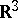, cf. also Link).

1) The Kauffman bracket skein module is based on the Kauffman bracket skein relation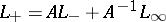, and is denoted by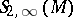. Among the Jones-type skein modules it is the one best understood. It can be interpreted as a quantization of the coordinate ring of the character variety of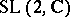representations of the fundamental group of the manifold, [a4], [a2], [a16]. For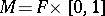, the Kauffman bracket skein module is an algebra (usually non-commutative). It is a finitely-generated algebra for a compact[a3], and has no zero divisors [a16]. Incompressible tori and two-dimensional spheres inyield torsion in the Kauffman bracket skein module; it is a question of fundamental importance whether other surfaces can yield torsion as well.

2) Skein modules based on the Jones–Conway relation (Homflypt relation) are denoted by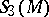and generalize skein modules based on the Conway relation which were hinted at by J.H. Conway. For,is a Hopf algebra (usually neither commutative nor co-commutative), [a19], [a11].is a free module and can be interpreted as a quantization [a6], [a18], [a10], [a19] (cf. also Drinfel'd–Turaev quantization).is related to the algebraic set ofrepresentations of the fundamental group of the manifold, [a17].

3) The skein module based on the Kauffman polynomial relation is denoted by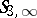and is known to be free for.

4) In homotopy skein modules,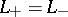for self-crossings. The best studied example is the-homotopy skein module with the skein relation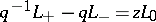for mixed crossings. Forit is a quantization, [a7], [a19], [a15], and as noted by U. Kaiser they can be almost completely understood using Lin's singular tori technique [a20].

5) The only studied skein module based on relations deforming-moves to date (2000) is the fourth skein module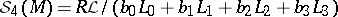, with possible additional framing relation. It is conjectured that inthis module is generated by trivial links. Motivation for this is the Montesinos–Nakanishi three-move conjecture (cf. Montesinos–Nakanishi conjecture).

6) Extending the family of knots,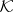, by singular knots, and resolving singular crossing byallows one to define the Vassiliev–Gusarov filtration: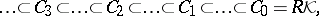where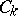is generated by knots withsingular points. Theth Vassiliev–Gusarov skein module is defined to be a quotient: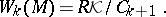The completion of the space of knots with respect to the Vassiliev–Gusarov filtration,, is a Hopf algebra (for). Functions dual to Vassiliev–Gusarov skein modules are called finite type or Vassiliev invariants of knots, [a12].

Skein modules have their origin in the observation by J.W. Alexander [a1] that his polynomials of three links,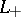,and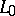in, are linearly related. They were envisioned by Conway (linear skein) [a5] and the outline of the theory was given first in the spring of 1987 [a9] after Jones' construction of his polynomial (the Jones polynomial) in 1984; see [a8], [a13], [a14] for the history of the development of skein modules. V.G. Turaev pointed out the importance of skein modules as quantizations, [a19] (cf. also Drinfel'd–Turaev quantization).

How to Cite This Entry:
Skein module. Encyclopedia of Mathematics. URL: http://encyclopediaofmath.org/index.php?title=Skein_module&oldid=16183
This article was adapted from an original article by Jozef Przytycki (originator), which appeared in Encyclopedia of Mathematics - ISBN 1402006098. See original article